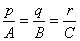§4  Lines and planes in space

The direction of the straight line

 name and description graphics [ direction angle ]        The angle a , b , g of the straight line OM passing through the origin O and the three coordinate axes is called the direction angle of the line ( the direction of OM is the direction away from the origin O ) :a = ∠ MOx , b = ∠ MOy , g = ∠ MOz [ direction cosine ]        The cosine of the direction angle of a line is called the direction cosine: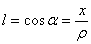, ,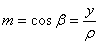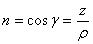In the formula , l 2 + m 2 + n 2 = 1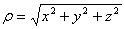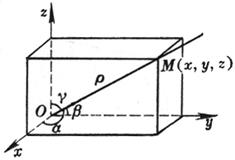[ Number of directions ]        The coordinates ( p , q , r ) of any point W on the straight line OM passing through the origin and parallel to the straight line L are called the number of directions of the straight line L, and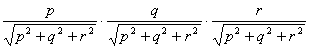is the direction cosine of the straight line OM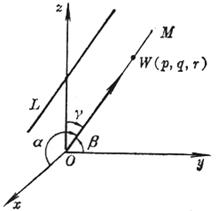name and description graphics [ Direction cosine of a line passing through two points ]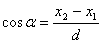, ,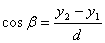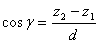in the formula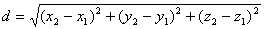At this time, the positive direction of the straight line is the direction from M 1 ( x 1 , y 1 , z 1 ) to M 2 ( x 2 , y 2 , z 2 ) .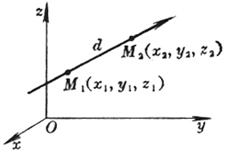The equation of the plane

 Equations and Graphics Description [ intercept ]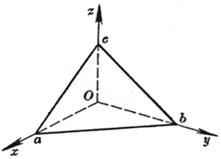a, b, c are called the intercepts of the plane on the three coordinate axes, respectively [ dot French ]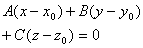( A , B , C are not equal to zero at the same time )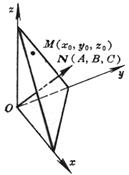The plane passes through the point M ( x 0 , y 0 , z 0 ) and the number of directions of the normal N is A , B , C [ Three-point type ]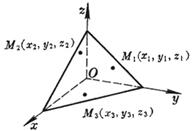The plane passes through three points :          M 1 ( x 1 , y 1 , z 1 )          M 2 ( x 2 , y 2 , z 2 )          M 3 ( x 3 , y 3 , z 3 ) or=0 equation with graphics Description [ General formula ]   Ax + By + Cz + D = 0   ( A , B , C are the direction numbers of the normal of the plane, and are not equal to zero at the same time )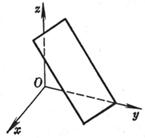When D = 0 , the plane passes through the origin        When A = 0 ( or B = 0 , or C = 0) , the plane is parallel to the x - axis ( or y - axis, or z - axis )        When A = B =0 ( or A = C =0 , or B = C =0) , the plane is parallel to the Oxy plane ( or Ozx , or Oyz ) [ normal type ]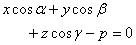( a , b , g are the direction angles of the normal line of the plane, p 3 0 is the length of the normal line, that is, the distance from the origin to the plane )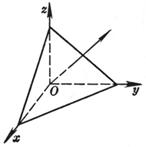The general formula of the plane can be transformed into the normal formula , which is called the normalization factor of the plane. When D < 0 , the positive sign is taken; when D > 0 , the negative sign is taken.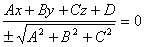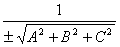[ vector ] ( r - r 0 ) × a = 0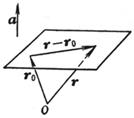The plane passes through the end point of the vector radius r 0 and is perpendicular to the known vector a , r is the vector radius of any point on the plane

The equation of a straight line

 Equations and Graphics Description [ General formula ( or face-to-face )] L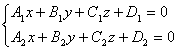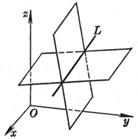Taking the straight line L as the intersection of two planes, its number of directions is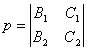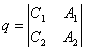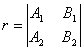[ Symmetrical ( or parametric )]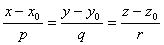or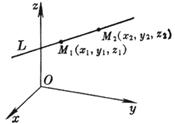The straight line L passes through the point M ( x 0 , y 0 , z 0 ) and has the number of directions p , q , r Equations and Graphics Description [ two-point type ]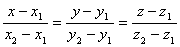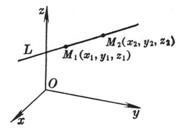The straight line L passes through two points M 1 ( x 1 , y 1 , z 1 ) and M 2 ( x 2 , y 2 , z 2 ) [ projective ] L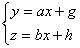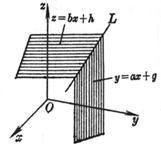The straight line L is the intersection of the two planes y = ax + g and z = bx + h ; it passes through the point (0, g , h ) and has direction numbers 1, a , b [ vector ]   r = r 0 + t a   (- ￥ < t < ￥ )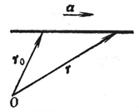The straight line L passes through the end point of the vector radius r 0 and is parallel to the known vector a , where r is the vector radius of any point on L

4.          Interrelationship between points, lines and planes in space

 Equations and Graphics Formula and Explanation [ Included angle between two planes ]  P 1 A 1 x + B 1 y + C 1 z + D 1 = 0  P 2 A 2 x + B 2 y + C 2 z + D 2 = 0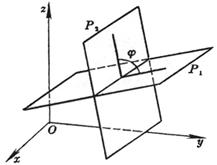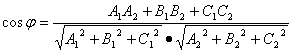where is the dihedral angle of the two planes P 1 and P 2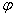Equations and Graphics Formula and Explanation [ Condition of plane bundle × collinearity of three planes ] P l    ( A 1 x + B 1 y + C 1 z + D 1 ) + l  ( A 2 x + B 2 y + C 2 z + D 2 ) = 0   ( l is a parameter, - ￥ < l < ￥ )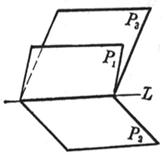[ Condition of plane handle × four planes co-point ] P l m     ( A 1 x + B 1 y + C 1 z + D 1 ) +       l ( A 2 x + B 2 y + C 2 z + D 2 ) +      m ( A 3 x + B 3 y + C 3 z + D 3 )       = 0   ( l , m are two independent parameters,    - ￥ < l , m < ￥ )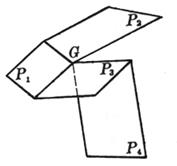For a definite value of l, P l represents an intersection of two planes P 1 and P 2 The plane of the line L , when l takes all values, the whole of the plane represented by P l passing through L is called the plane beam, and L is called the axis of the beam .        Let P 3 be A 3 x + B 3 y + C 3 z + D 3 = 0 , then the condition for the collinearity of the three planes P 1 , P 2 , P 3 is a matrixThe rank of is equal to 2.                 For a pair of definite values ​​of l , m , P l m represents a plane passing through the intersection G of the three planes P 1 , P 2 and P 3 , when l , m take all values, P l m represents the plane passing through G The whole of is called the plane handle, and G is called the vertex of the handle .              Assuming that P 4 is A 4 x + B 4 y + C 4 z + D 4 = 0 , the condition for the common points of the four planes P 1 , P 2 , P 3 , and P 4 is the determinant[ distance between points and faces ]   normal   x cos a + y cos b + z cos g - p = 0 General formula Ax + By + Cz + D = 0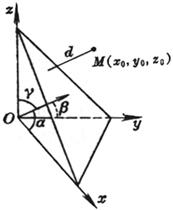d method = | x 0 cos a + y 0 cos b + z 0 cos g - p |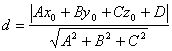where d is the distance from point M ( x 0 , y 0 , z 0 ) to the plane Equations and Graphics Formula and Explanation [ distance of dotted line ] L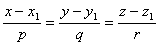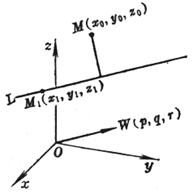where d is the distance from the point M ( x 0 , y 0 , z 0 ) to the straight line L , i , j , k are the unit vectors on the three coordinate axes, and the outermost symbol “ | | ” represents the modulus of the vector [ Included angle between two straight lines ] L 1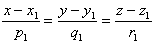L 2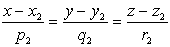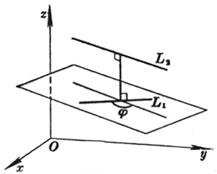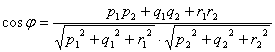where j is the angle between the two straight lines L 1 and L 2 [ The shortest distance between two non-parallel lines ]        L 1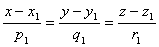L 2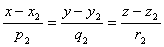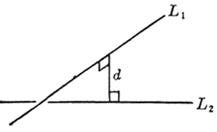The so - called shortest distance refers to the distance between the common vertical line of L 1 , L 2 and the intersection of the two lines . The equation of the plane isEquations and Graphics Formula and Explanation [ The angle between the line and the plane ] LP Ax + By + Cz + D = 0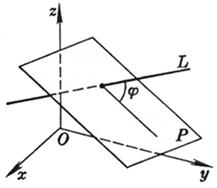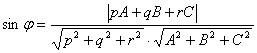where j is the angle between the straight line L and the plane P

[ Parallel and perpendicular conditions of straight lines and planes ]

 parallel condition vertical condition line to line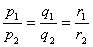face to face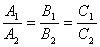line and surface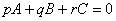p 1 p 2 + q 1 q 2 + r 1 r 2 = 0          A 1 A 2 + B 1 B 2 + C 1 C 2 = 0